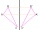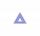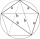# Pythagorean theorem - math word problems

#### Number of problems found: 704

• An observerAn observer standing west of the tower sees its top at an altitude angle of 45 degrees. After moving 50 meters to the south, he sees its top at an altitude angle of 30 degrees. How tall is the tower?
• Touch x-axisFind the equations of circles that pass through points A (-2; 4) and B (0; 2) and touch the x-axis.
• Pyramid 8Calculate the volume and the surface area of a regular quadrangular pyramid with the base side 9 cm and side wall with the base has an angle 75°.
• Vector 7Given vector OA(12,16) and vector OB(4,1). Find vector AB and vector |A|.
• Cone containerRotary cone-shaped container has a volume 1000 cubic cm and a height 12 cm. Calculate how much metal we need for making this package.Calculate the surface area and volume of a regular quadrangular pyramid: sides of bases (bottom, top): a1 = 18 cm, a2 = 6cm angle α = 60 ° (Angle α is the angle between the side wall and the plane of the base.) S =? , V =?
• Calculate 8Calculate the coordinates of point B axially symmetrical with point A[-1, -3] along a straight line p : x + y - 2 = 0.
• Pile of sandA large pile of sand has been dumped into a conical pile in a warehouse. The slant height of the pile is 20 feet. The diameter of the base of the sand pile is 31 feet. Find the volume of the pile of sand.
• Hexagonal pyramidFind the volume of a regular hexagonal pyramid, the base edge of which is 12 cm long and the side edge 20 cm.
• Medians and sidesDetermine the size of a triangle KLM and the size of the medians in the triangle. K=(-5; -6), L=(7; -2), M=(5; 6).
• Five-gonCalculate the side a, the circumference and the area of the regular 5-angle if Rop = 6cm.
• The regularThe regular triangular prism has a base in the shape of an isosceles triangle with a base of 86 mm and 6.4 cm arms, the height of the prism is 24 cm. Calculate its volume.
• SailboatThe 20 m long sailboat has an 8 m high mast in the middle of the deck. The top of the mast is fixed to the bow and stern with a steel cable. Determine how much cable is needed to secure the mast and what angle the cable will make with the ship's deck.
• Triangular prismThe triangular prism has a base in the shape of a right triangle, the legs of which is 9 cm and 40 cm long. The height of the prism is 20 cm. What is its volume cm3? And the surface cm2?
• A concrete pedestalA concrete pedestal has a shape of a right circular cone having a height of 2.5 feet. The diameter of the upper and lower bases are 3 feet and 5 feet, respectively. Determine the lateral surface area, total surface area, and the volume of the pedestal.
• Plane IIA plane flew 50 km on a bearing 63degrees20 and the flew on a bearing 153degrees20 for 140km. Find the distance between the starting point and the ending point
• Rotary bodiesThe rotating cone and the rotary cylinder have the same volume 180 cm3 and the same height v = 15 cm. Which of these two bodies has a larger surface area?
• Truncated cone 6Calculate the volume of the truncated cone whose bases consist of an inscribed circle and a circle circumscribed to the opposite sides of the cube with the edge length a=1.
• The hemisphereThe hemisphere container is filled with water. What is the radius of the container when 10 liters of water pour from it when tilted 30 degrees?
• On a lineOn a line p : 3 x - 4 y - 3 = 0, determine the point C equidistant from points A[4, 4] and B[7, 1].

Do you have an interesting mathematical word problem that you can't solve it? Submit a math problem, and we can try to solve it.

We will send a solution to your e-mail address. Solved examples are also published here. Please enter the e-mail correctly and check whether you don't have a full mailbox.

Please do not submit problems from current active competitions such as Mathematical Olympiad, correspondence seminars etc...

Pythagorean theorem is the base for the right triangle calculator.